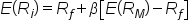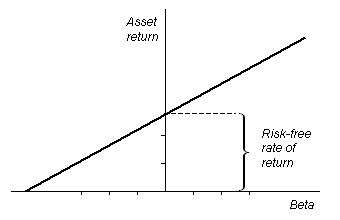# Understanding the Security Market Line

Author: Sophia Tutorial
##### Description:

Analyze a security's position on a graph of the security market line.

(more)### Developing Effective Teams

*No strings attached. This college course is 100% free and is worth 1 semester credit.

37 Sophia partners guarantee credit transfer.

299 Institutions have accepted or given pre-approval for credit transfer.

* The American Council on Education's College Credit Recommendation Service (ACE Credit®) has evaluated and recommended college credit for 33 of Sophia’s online courses. Many different colleges and universities consider ACE CREDIT recommendations in determining the applicability to their course and degree programs.

Tutorial

what's covered
In this lesson, you will learn about a security's position on a graph of the security market line. Specifically, this lesson will cover:
1. Expected Risk and Risk Premium
2. Defining the Security Market Line
3. Impact of the SML on the Cost of Capital

## 1. Expected Risk and Risk Premium

A certain amount of risk is inherent in any investment. Risk can be defined, generally, as the potential that a chosen action or activity (including the choice of inaction) will lead to a loss or an undesirable outcome. The notion of risk implies that a choice having an influence on the outcome exists. More specifically to finance, risk can be seen as relating to the probability of uncertain future events. In return for undertaking risk, investors expect to be compensated in such a way as to reasonably reward them. This is a central theme in the subject of finance. In the financial realm, two types of risk exist:

• Systemic risk is the risk associated with an entire financial system or entire market. This type of risk is inherent in all marketable securities and cannot be diversified away.
• Unsystematic risk is risk to which only specific classes of securities or industries are vulnerable. This type of risk is uncorrelated with broad market returns, and with proper grouping of assets can be reduced or even eliminated. Because of this characteristic, investors are not rewarded for taking on unsystematic risk.
Systematic risk can be understood further using the measure of beta. This is a number describing the correlated volatility of an asset in relation to the volatility of the benchmark that said asset is being compared to — usually the market as expressed in an index.

Values of beta can be interpreted using the following information:

Values of Beta Description
Beta less than 0 Asset generally moves in the opposite direction as compared to the index.
Beta equal to 0 Movement of the asset is uncorrelated with the movement of the benchmark.
Beta between 0 and 1 Movement of the asset is generally in the same direction as, but less than, the movement of the benchmark.
Beta equal to 1 Movement of the asset is generally in the same direction as, and about the same amount as, the movement of the benchmark.
Beta greater than 1 Movement of the asset is generally in the same direction as, but more than, the movement of the benchmark.

The term market risk premium refers to the amount by which an asset’s expected rate of return exceeds the risk-free rate. The difference between the return of an asset in question and that of a risk-free asset — for instance, a US Treasury bill — can be interpreted as a measure of the excess return required by an investor on the risky asset. The risk premium, along with the risk-free rate and the asset’s beta, is used as an input in popular asset valuation techniques, such as the Capital Asset Pricing Model.

terms to know

Beta (β)
A number describing the correlated volatility of an asset in relation to the volatility of the benchmark that said asset is being compared to.
The amount by which expected rate of return of the exchange system exceeds the risk-free interest rate.
Capital Asset Pricing Model (CAPM)
An equation that assesses the required rate of return on a given investment based upon its risk relative to a theoretical risk-free asset.

## 2. Defining the Security Market Line

The security market line, also known as the “characteristic line,” is the graphical representation of the capital asset pricing model. It is a hypothetical construct based on a world of perfect information. In the absence of perfect information, we can more or less assume historical data will give us an accurate expectation of what kind of returns and risk to expect with a particular investment of capital. The security market line graphs the systematic, non-diversifiable risk (stated in terms of beta) versus the return of the whole market at a particular time, and shows all risky marketable securities.

The security market line is defined by the equation:

formula
Security Market LineLook at the equation and remember that old formula of a line: y = mx + b. In this case, it looks rearranged, like y = b + mx. But the real question is, what do the slope and y-intercept actually represent?
The y-intercept in the secure market line, or where the vertical axis meets the horizontal axis, is also known as the risk-free rate. The assets that are plotted above the line are said to be undervalued for a given level of risk. Conversely, the assets plotted below the line are said to be overvalued due to the given level of risk.

The slope of the SML is the premium that the market charges for risk.The idea of a security market line follows from the ideas asserted in the last section, which is that investors are naturally risk-averse, and a premium is expected to offset the volatility of a risky investment. In a perfect world, with perfect information, any capital investment is on the security market line. The idea of a security market line is important for understanding the capital asset pricing model.

terms to know

Security Market Line (SML)
The representation of the capital asset pricing model. It displays the expected rate of return of an individual security as a function of systematic, non-diversifiable risk (its beta).
Risk-Free Rate
The theoretical rate of return of an investment with no risk of financial loss.

## 3. Impact of the SML on the Cost of Capital

The security market line is a graphical representation of the capital asset pricing model that illustrates the idea that investments are priced efficiently based on the expected return and beta value (risk). Companies often turn to capital markets in order to generate funds — using the issuance of either debt or equity. The cost of obtaining funds in such a manner is known as a company’s cost of capital. There is a tradeoff between a security’s price and its expected return. If the price of the instrument goes up, its expected returns go down, and vice versa. A firm that is raising capital would like to sell these instruments for a high price, and investors want to buy them for a low price.

The location of a financial instrument above, below, or on the security market line will lead to consequences for a company’s cost of capital:

• An instrument plotted below the SML would have a low expected return and a high price. This market situation would be quite attractive from the perspective of a company raising capital; however, such an investment wouldn’t make sense for a rational buyer. The rational investor will require either a higher return or lower price, which will both result in a higher cost of capital for the company.
• An instrument plotted above the line has a high expected return and a low price. This would not be an attractive market situation for a company looking to raise capital. Such a firm wants to raise as much money as possible, which means getting investors to pay the highest price possible.
• An instrument plotted on the SML can be thought of to be fairly priced for the amount of expected return. Such an instrument would be a fair investment from an individual’s perspective, and would lead to a fair cost of capital from a company’s perspective.
summary
In this lesson, you continued to learn about expected risk and the concept of the risk premium, or the amount by which an asset’s expected rate of return exceeds the return on a risk-free asset like a U.S. Treasury bill. The risk premium can be plotted along the security market line, which is a graphical representation of the capital asset pricing model. The impact of the security market line on the cost of capital is such that an instrument plotted above the line is attractive to the issuing company but not to an investor. The reverse is true for instruments plotted below the line.

Best of luck in your learning!

Source: THIS CONTENT HAS BEEN ADAPTED FROM LUMEN LEARNING'S “Understanding the Security Market Line” TUTORIAL.

Terms to Know
Beta (β)

A number describing the correlated volatility of an asset in relation to the volatility of the benchmark that said asset is being compared to.

Capital Asset Pricing Model (CAPM)

An equation that assesses the required rate of return on a given investment based upon its risk relative to a theoretical risk-free asset.

The amount by which expected rate of return of the exchange system exceeds the risk-free interest rate.

Risk-Free Rate

The theoretical rate of return of an investment with no risk of financial loss.

Security Market Line (SML)

The representation of the capital asset pricing model. It displays the expected rate of return of an individual security as a function of systematic, non-diversifiable risk (its beta).

Formulas to Know
Security Market LineRating